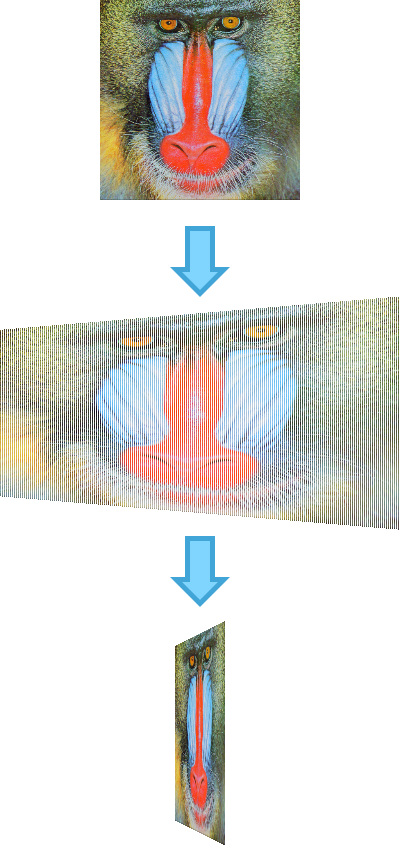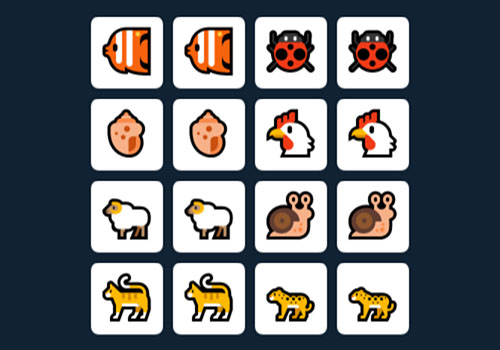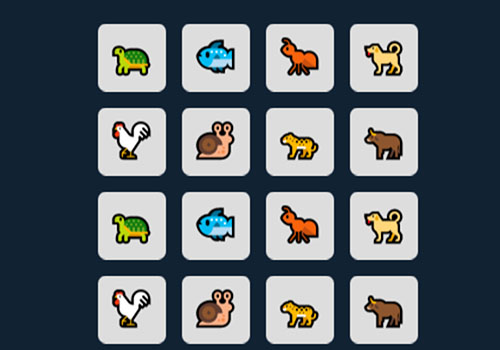# JS1K 2017 part 1: Memoji

Last year I didn’t have the time to participate in JS1K… but this one I could! I made two games, so this article describes the creation process of the first one: Memoji, a memory game.

## Preface

Back in 2012, the theme for JS1K was “Love”. My first idea was to make a memory game, to be named “Loving memories”.

I thought that it would be trivial to implement rotating cards using trapezoids to simulate a 3D effect, but when implementing the prototype, I learned that, unfortunately, HTML5 Canvas lacks that sort of transformation, as it’s limited to scaling, translating, rotating, and skewing.

That didn’t stop me, and I found a way to achieve the same effect: rendering an image by painting it one column at a time at different heights, using the `drawImage(image, sx, sy, sWidth, sHeight, dx, dy, dWidth, dHeight);` method.While this works, and it’s quick enough, the resulting function takes quite a bit of the available space. At that moment, I didn’t think it would be possible to implement the game the way I wanted, so I gave up and made a different one, which I’m still quite proud of.

Fast forward to 2017! I decided to revisit my concept and see if it was feasible. I could see very soon that it was! When I first investigated it, I didn’t know about Regpack, or canvas literals hashing, and this year’s rules also allow ES6, so it was quite clear that it would fit.

Hence, I decided to go for it, but I also set my goal to make it a polished game; many times, because of the space contraints, developers need to find shortcuts, like using an static size, or placing the game at the (0,0) coordinates to simplify the code, but since the game itself is quite simple, I wanted to provide a proper experience in both desktop and mobile.

## Enablers

As I mentioned earlier, before being able to implement this game, some things had to be developed, and I had to learn a few tricks:

• Regpack: this is a very interesting tool, which is used by most JS1K demos. It has evolved quite a bit through the years, adding new features. Basically, it will take some code, analizes it, find repeated strings, and generates a code that will expand into the original version. The higher number of repeated strings, the better the compression will be, so the code can be fine tuned to take advantage of it. For instance, it’s a good idea to minimize the number of used variables as much as possible, and also try to reuse the same patterns over and over.
• Color emojis: During the past few months there has been quite an advancement in the adoption of color emojis by desktop browsers (as they have been available in cell phones for quite a bit). Firefox now has an embedded color emoji font in all platforms, and both Mac and Windows now come with beautiful, full color emojis. For a space-constrained competition like JS1K, that’s a treasure chest that’s difficult to ignore, so this year a large number of entries have decided to take advantage of that feature.
• Canvas literal hashing: one important handicap of using JavaScript in demos is that the JavaScript API literals are quite long; strings like `globalCompositeOperation` or `requestAnimationFrame` really fill up the space quickly! A technique that has been used to work around that is to “compress” all strings in the Canvas object using a function like this:
``````for(i in c)c[i+[i]]=i;
``````

After this function is applied, we can access `c.globalAlpha` as `c[c.gA]`, or `c.createLinearGracient` as `c[c.cL]`. The only issue with this approach is that the game may stop working if new functions are added to the Canvas that collide with the existing mappings… but it works today!

## Tricks

In order to make everything fit in just 1024 bytes, it’s important to analyze the code and find alternatives that do a the same job (or as close as possible) while consuming less bytes (or reuse existing code so it can be compressed better). Here’s a few that went into this game:

• Emoji selection: From the Unicode code point U+1F400 (rat 🐀) to U+1F43F (chipmunk 🐿) we can find the animal emojis, which are rendered in color where available. Those characters are quite adequate for this sort of game, as they’re quite different from each other, and they look quite good! For the game we need to pick 8 random animals, so in order not to use the same animal twice, the following code is used:
``````figure+=1+Math.random()*7|0;
``````

While it’s not uniformly distributed (low number characters will be used more often than high ones), it fully prevents duplicates without using many bytes.

• Card randomization: in order to randomly place the different cards on the board, I decided to use the following code snippet:
``````board.sort(()=>.5 - Math.random());
``````

I really like this solution because we let the JS engine do the heavy lifting. Unfortunately, this is not purely random, and it’s specially inefficient on the edges… which was a problem with my default distribution:There was a high chance that the first two cells and the last two weren’t altered, which was quite noticeable after playing for a bit, so with a few extra bytes I altered the default layout to the following:It may not look like a big difference, but it works a lot better with the randomized sorting.

• Card shading: in order to make the 3D rotating effect look better, some shading was added to the resulting image. As explained before, each column is drawn individually, so a black rectangle is painted over it with an alpha channel value calculated from the rotation angle. This creates some artifacts around the cards’s rounded corners, so in order to make the problem less noticeable, the animation speed became “ease in out”, which also improves the 3D feeling of the cards.

## Commented source code

``````// Hashing function
R = function(e,n,Z){
for(Z in e)e[Z+(Z[n||6])]=e[Z]&&e[Z].call?e[Z]:Z;
};

R(c);
R(a,3);
w = a[a.wt], h=a[a.hg];

// Calculate side
s = (((w<h)?w:h)>>2)-24;

// Pseudo-3D function
S = function(img, left, top, portviewWidth, n){
q = n;
if (portviewWidth-s) {
c[c.fy] = "#000";
for(n = portviewWidth; n--;) {
c.da(img,
n * s /portviewWidth,
0,
s / portviewWidth,
s,
left + n + (s-portviewWidth>>1),
top - .5 * q * (s-portviewWidth) * (n - portviewWidth/2)/(3*portviewWidth),
1,
s + q * (s-portviewWidth) * (n - portviewWidth/2) / (3*portviewWidth));
// Frame darkening
c[c.gA]=.7*(1-portviewWidth/s);
c.fc(
left + n + (s-portviewWidth>>1),
top - .5 * q * (s-portviewWidth) * (n - portviewWidth/2)/(3*portviewWidth),
1,
s + q * (s-portviewWidth) * (n - portviewWidth/2) / (3*portviewWidth));
c[c.gA] = 1;
}
} else {
// Efficient painting path
c.da(img,left,top);
}
}

g = [];
// Store canvas object
q = c;
f = -1;

// Initialize board
for(n=17;n--;){
// New canvas
a=a.cn()
R(a,8);
// Reuse canvas for better RegPack compression
c=a.gx("2d");
R(a,3);
// Cell value
a.f=f=(n<8)?g[n+8].f:(f+1+Math.random()*7|0);
// Set size (if not set, it's very slow on mobile)
a[a.wt]=a[a.hg]=s;
// Canvas reduction
R(c);

// Pick colors depending of the card type: normal or back
c[c.sS]=(n-16)?"#ddd":"#553";
c[c.fy]=(n-16)?"#ddd":"#aa7"
// Round box
c[c.li]="round";
c[c.ld]=16;

// Stroke
c.sR(8,8,s-16,s-16);
// Fill Rect
c.fc(8,8,s-16,s-16);

if (n-16){
c[c.fy]="#000"
c.font=s/2 + "px d";
c[c.ti]="center"
c[c.ts]="middle"
// emojis to use: 61 from 1F400 (128e3)
c.fx(a.y=String.fromCodePoint(f+128e3),s/2,s/2)
}
// Store it as a board cell or the card back
(n-16)?g[n]=a:B=a;
}

// Restore canvas object
c = q;

// Randomize
g.sort(function(){return.5 - Math.random()});

A=function(W,X,Y,Z,n){
// Clean the canvas
c[c.fy] = "#123"
c.fc(0,0,w,h)

// Paint all cells
for (n=16;n--;) {
S(((g[n].z>1&&g[n].x<13)||(g[n].z&1&&g[n].x>13)||g[n].z&4)?g[n]:B,
w/2-s*2+(s+16)*(n%4)-16,
h/2-s*2+(s+16)*(n/4|0)-16,
(g[n].x&&--g[n].x&&g[n].z-4)?s*Math.abs((g[n].x-12)/12)|0:s,
((g[n].z&1?-1:1)*(g[n].x<13?-1:1)));
if (!g[n].x&&g[n].z&4) {
g[n].z = 1;
g[n].x = 24;
}
}

// Are we done animating?
if (k>-1 && !g[k].x && !g[f].x) {
// Do we have a pair?
if (g[f].y == g[k].y) {
g[f].z = g[k].z = 3;
} else {
g[f].z = g[k].z=4;
g[f].x = g[k].x=24;
}
// Reset selections
k = f = -1;
}
requestAnimationFrame(A);
};

// Initialize
A(k = f = -1);

// Mouse click listener
onclick = function(e,clickedX,clickedY){
// Store clicked row & column
clickedX = (e.pageX-w/2+s*2+16)/(s+16)|0;
clickedY = (e.pageY-h/2+s*2+16)/(s+16)|0;
if (clickedX > -1
&&clickedX<4
&&clickedY>-1
&&clickedY<4
&&g[e = clickedX+4*clickedY].z!=3
&&e-f
&&k<0) {
(f>-1)?k = e:f = e;

// Animation
g[e].x = (g[e].z&4)?0:g[e].x||24;
// 2 is opening animation
g[e].z = 2;
}
};

// Processing the release version:
// - Minify with Google Closure Compiler (simple optimizations)
// - Remove final semicolon
// - Change functions to arrow functions, reuse function signature
// - Change getContext to use backticks (ES6)
// - Fix loops to use same index variable
// - Pack with Regpack
``````

## Final words

While there’s nothing extraordinary going on with this memory game, I’m really happy with the end result, and most importantly, to have been able to achieve what I thought was impossible a few years ago. Plus, my daughters love to play with it in my cell phone 😄